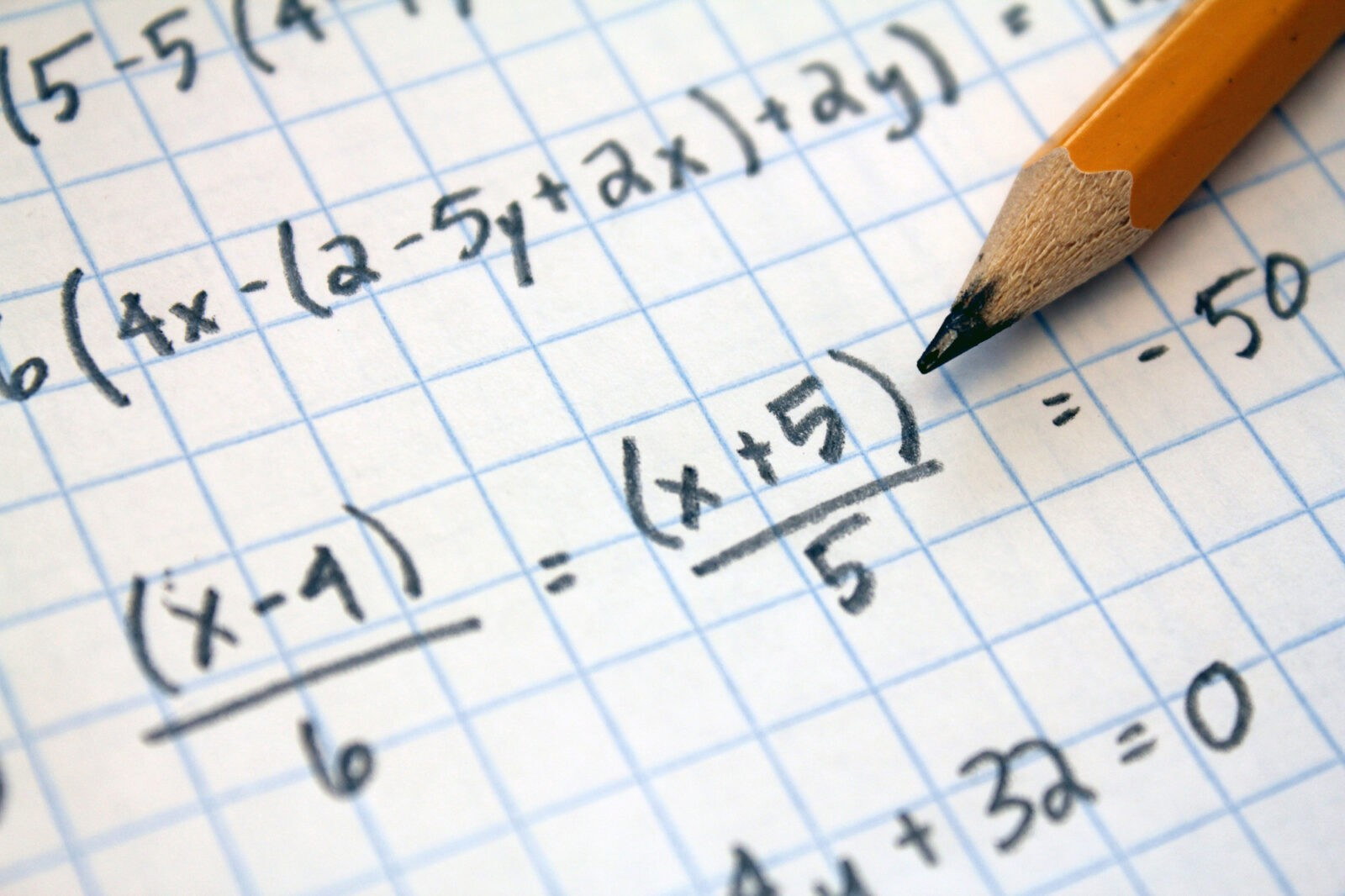# Algebra Explained- Why Do We Need to Factor EquationsAre you good at math? Do you love it? Does the idea of an equation make you want to spin around in circles of joy?

If you’re like most of the world, you thought, “heck no!”. That’s okay. We won’t tell you that math is fun, but we are going to tell you the reasons behind it.

The reasons exist and they aren’t there to just confuse you. What specifically are we/you confused about today? Why we factor polynomials.

In essence, we factor them to translate them into more workable terms. You take complex looking algebra equations and turn it into something easier.

Yes, it’ll still have variables, but you can see them written out. Learn a little bit more about why and how to factor them below.

## Why Do We Factor?

Like we talked about above, factoring is really translating something into a simpler form.

Instead of looking at something that’s x squared and getting scared by the exponent, we can look at it as x times x. If someone said 14^2, would you know that right off the top of your head?

No, but if someone said 14 x 14, now that’s a simple multiplication problem. It’s the same thing and you’ll get the same answer, but one is less intimidating.

## How to Factor

Now that you know learning how to factor will make your life easier, are you more willing to do it?

You have the equation, x^2 + 5x + 6

What do you see here? First off, put a one in front of the singular X. If you ever see a lone X, there’s a one in front of it – math is just lazy.

Now that you’ve done that, draw two sets of parentheses. Two, because it’s x squared and squared means two.

( x _ )(x _ ) is set up under our equation. Now our job is to figure out what other numbers go in the parentheses.

The two missing numbers need to multiply together to give us our constant (number with no variable) 6. What two numbers multiply to give us 6?

1 and six would work, except the same numbers that have to multiply to six, have to add or subtract to equal our middle number, five x.

So, we’re left with three and two. Multiplied together it makes six but added they make five.

So now our equation looks like (X+2)(X+3). Notice that we didn’t have an equals sign in our original equation, so simplifying is all we can do. You’re done once it’s in the above factor form.

## The Other Way Around

If you were told to show the equation for (X+2)(X+3) what would you do? Notice we used the same equation from above for simplicity.

Well, you use FOIL. First, Outer, Inner, Last. Stick with us, this isn’t meaningless, we promise.

First means the first number in the first parentheses times the first number in the second parentheses.

That would be x and x, from our equation. X times X equals, X, squared.

So we mark that down. X squared, what’s next? Do the outer in foil. Take the first x in the first parentheses and multiply it times the last number in the second set.

This is x times 3, which is three x. Now we have X^2 + 3X.

Now we do the inner – which are the two numbers where the middle of the parentheses meets. In our equation it’s 2 and X. We multiply them and get 2x.

So now we have X^2 + 2x + 3x.

Finally, we only have one set left, the last. We take the same 2 from our first set of parentheses and multiply it by our last number, which is three.

Two times three is six.

Now we have X^2 + 2x + 3x +6.

### Combine

Now that we have our equation and the parentheses are gone, what do we do? Look for numbers we can combine. What looks similar in this problem?

Both 2x and 3x only have one x – so let’s add them to get 5x. With them combined, we’re back to our exact same starting equation.

X^2 + 5X + 6

We’re done – that’s as far as we can go, given we don’t have an equals sign.

Do you see how it’s the same equation and we get the same numbers backward and forwards? That’s the magic of factoring and FOIL.

To remind you, FOIL is first, outer, inner, last. Memorize it, sing it, dance it, do whatever works for you to remember it!

## Why Factor Algebra Equations?

Well, it’s not something you can do on a calculator. If you pay the big bucks, some computerized calculations have FOIL buttons. But those aren’t the type most people can take or use with tests.

So, you need to learn how to do it the old-fashioned way, with paper and pencil. If you’re lucky and you’re doing math with a computer around, and you’re allowed calculators, there are special online factoring options.

## Online Factoring Calculator

This online factoring calculator makes algebra equations seem handlable, if not easy. It’s not hard to figure out where to put which numbers, as some online input forms can be.

You simply type out your equation and hit solve. It’s that simple! We also offer a fraction calculator, for those times you really don’t want to find a common denominator.

Want a reminder or a lesson on how to calculate fractions? Does the sound of keep, switch, flip ring a bell? That’s the method for dividing fractions. Still not ringing a bell?

Don’t over-complicate things. Use our fraction calculator and you’ll be ready to go!

Algebra Explained- Why Do We Need to Factor Equations
Scroll to top